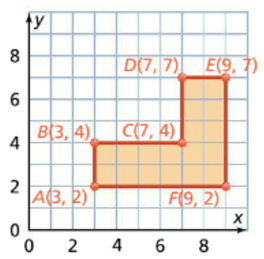Mathematics
Easy

Question

# Calculate the perimeter of the irregular figure## 20    22    18    10Hint:

## The correct answer is: 22

### The given coordinates of the figure are:A is (3,2)B is (3,4)C is (7,4)D is (7,7)E is (9,7)F is (9,2)We have to find the length of the all sidesTo find the length we will use the following criteria:1) If x coordinate is same, we will find the absolute distance using y coordinate.2) If y coordinate is same we will use the x coordinate to find absolute distance.3) If the points are in same quadrant, we will have to subtract the absolute distances to find length.4) If the points are in adjacent quadrants, we will have to add the absolute distances to find length.All points are in same quadrant.Side AB:For this, x coordinate is same. We will use y coordinate.Absolute distance of the point A = |2|Absolute distance of the point B = |4|Length of AB = |4| - |2|= 2Side BC:For this, y coordinate is same. We will use x coordinate.Absolute distance of the point B = |3|Absolute distance of the point C = |7|Length of BC = |7| - |3|= 4Side CD:For this, x coordinate is same. We will use y coordinate.Absolute distance of the point C = |4|Absolute distance of the point D = |7|Length of CD = |7| - |4|= 3Side DE:For this, y coordinate is same. We will use x coordinate.Absolute distance of the point D = |7|Absolute distance of the point E = |9|Length of DE = |9| - |7|= 2Side EF:For this, x coordinate is same. We will use y coordinate.Absolute distance of the point E = |7|Absolute distance of the point F = |2|Length of EF = |7| - |2|= 5Side FA:For this, y coordinate is same. We will use x coordinate.Absolute distance of the point F = |9|Absolute distance of the point A = |3|Length of FA = |9| - |3|= 6To find perimeter, we add all sides.Perimeter of the figure ABCDEFA = AB + BC + CD + DE + EF + FA= 2 + 4 + 3 + 2 + 5 + 6= 22 unitsTherefore, the perimeter of given figure is 22 units.

An absolute distance is the length of the point from the respective axis. If x coordinate is same then its length is measured from x axis. It is a positive quantity. We can use distance formula to find the length of sides of rectangle too.

### Related Questions to study#### With Turito Foundation.#### Get an Expert Advice From Turito.